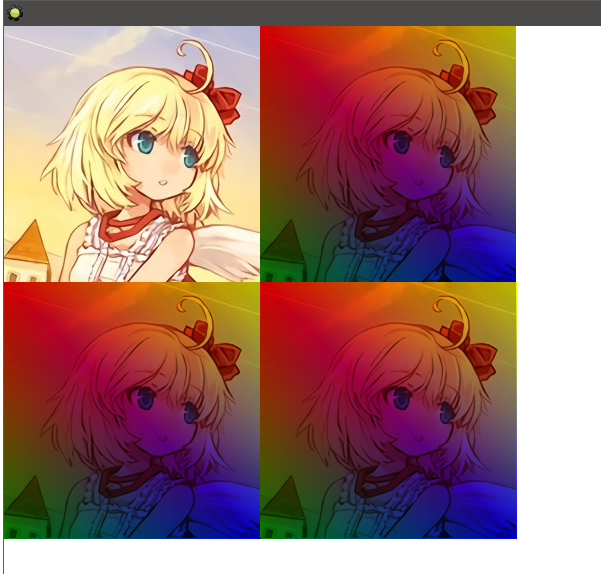## 颜色的混合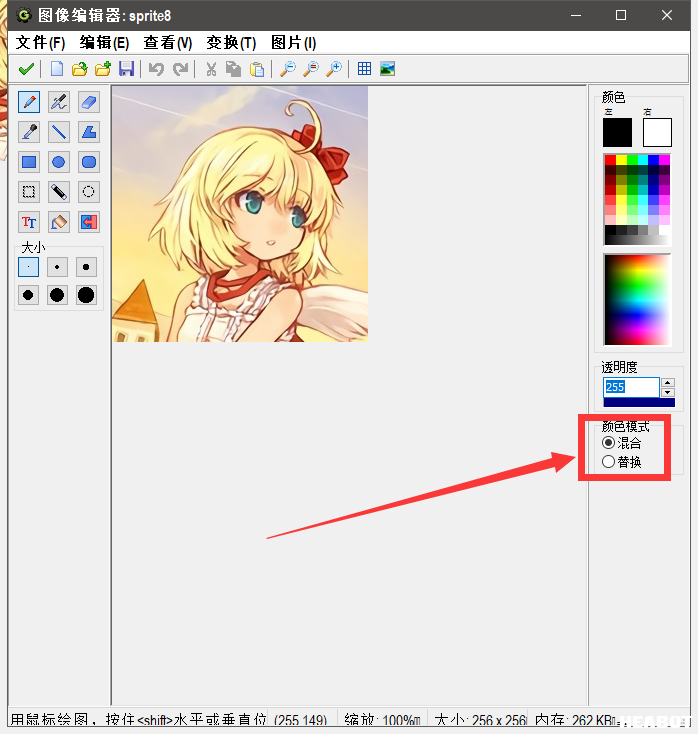Rd = 1, Gd = 0, Bd = 0, Ad = 0.47,

Rs = 0, Gs = 0, Bs = 1, As = 0.47,

Ro = 0.34, Go = 0, Bo = 0.65, Ao = 0.72

```1 2 3 4 Ao = As + Ad * (1 - As) Ro = (Rs * As + Rd * Ad * (1 - As)) / Ao Go = (Gs * As + Gd * Ad * (1 - As)) / Ao Bo = (Bs * As + Bd * Ad * (1 - As)) / Ao ```

## 混合因素

```1 2 3 4 Ro = Rs * a1 + Rd * b1 Go = Gs * a2 + Gd * b2 Bo = Bs * a3 + Bd * b3 Ao = As * a4 + Ad * b4 ```

GM8所提供的混合因素有：

• `bm_zero` 混合因素是 `(0, 0, 0, 0)`
• `bm_one` 混合因素是 `(1, 1, 1, 1)`
• `bm_src_color` 混合因素是 `(Rs, Gs, Bs, As)`
• `bm_inv_src_color` 混合因素是 `(1 – Rs, 1 – Gs, 1 – Bs, 1 – As)`
• `bm_src_alpha` 混合因素是 `(As, As, As, As)`
• `bm_inv_src_alpha` 混合因素是 `(1 – As, 1 – As, 1 – As, 1 – As)`
• `bm_dest_alpha` 混合因素是 `(Ad, Ad, Ad, Ad)`
• `bm_inv_dest_alpha` 混合因素是 `(1 – Ad, 1 – Ad, 1 – Ad, 1 – Ad)`
• `bm_dest_color` 混合因素是 `(Rd, Gd, Bd, Ad)`
• `bm_inv_dest_color` 混合因素是 `(1 – Rd, 1 – Gd, 1 – Bd, 1 – Ad)`
• `bm_src_alpha_sat` 混合因素是 `(f, f, f, 1)`; 其中 `f = min(As, 1 – Ad)`

## 设置混色模式

• `draw_set_blend_mode_ext(src, dest)` 这个函数用来设置混色模式。参数 src 填写 source 的混合因素，参数 dest 填写 destination 的混合因素。

• `draw_set_blend_mode(mode)` 这个函数是上面这个函数的简化版，参数只能选填 `bm_mormal``bm_add``bm_subtract`, `bm_max` 四个参数。

• `bm_normal` 对应 `(bm_src_alpha, bm_inv_src_alpha)`
• `bm_add` 对应 `(bm_src_alpha, bm_one)`
• `bm_subtract` 对应 `(bm_zero, bm_inv_src_color)`
• `bm_max` 对应 `(bm_src_alpha, bm_inv_src_color)`

### bm_normal

`bm_normal` 就是常规状态下，不设置混色模式时 GM8 的默认混合因素。

`bm_add` 是对颜色的加强，通常用于暗色调下去除黑色背景。比如你看中了一张素材图片，但是它的黑色底色很难去除：```1 2 3 draw_set_blend_mode(bm_add); draw_sprite(sprite_index, 0, x, y); draw_set_blend_mode(bm_normal); ```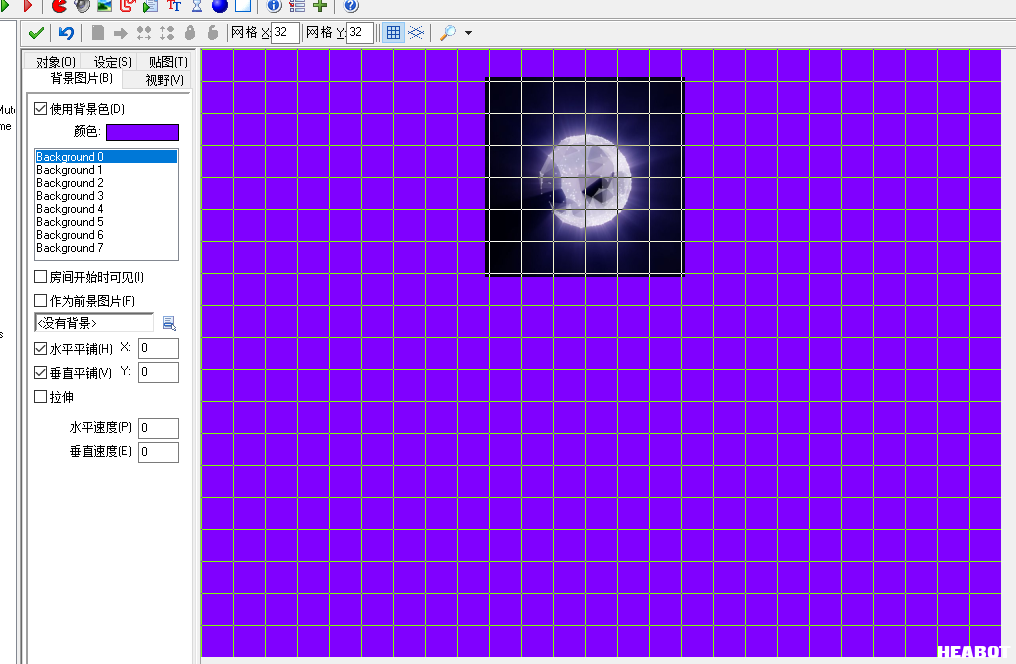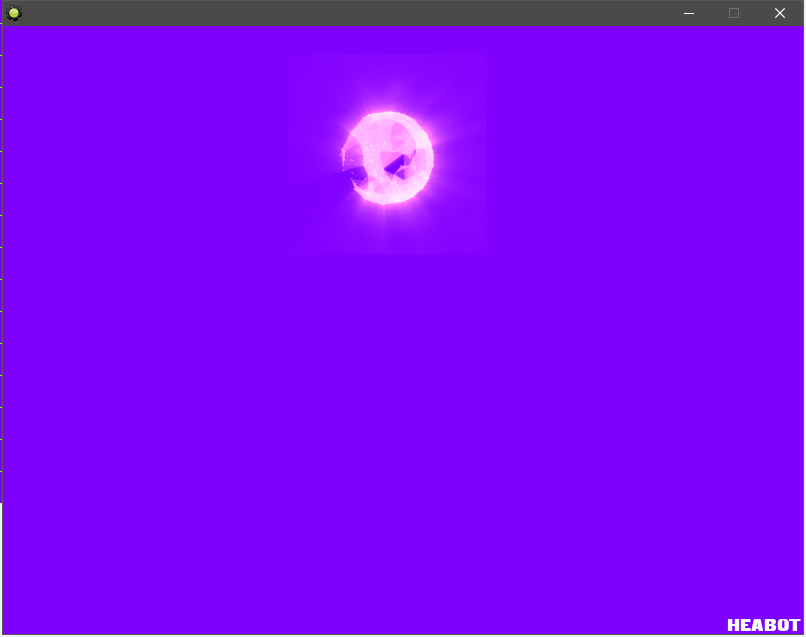### bm_subtract

`bm_subtract` 多用于表面（surface）之中，可以在表面上“挖洞”，这个我们日后再说。

### bm_max

`bm_max``bm_add` 效果比较接近，混合因素稍有不同，destination 的权重相比 `bm_add` 更低，因此单纯从去除黑色底色的功能来讲大概是要比 `bm_add` 更好一点点的（如下图所示），但是感觉大部分人似乎都更喜欢用 `bm_add`？不过 `bm_max` 仍然是不适合用在亮色背景下的。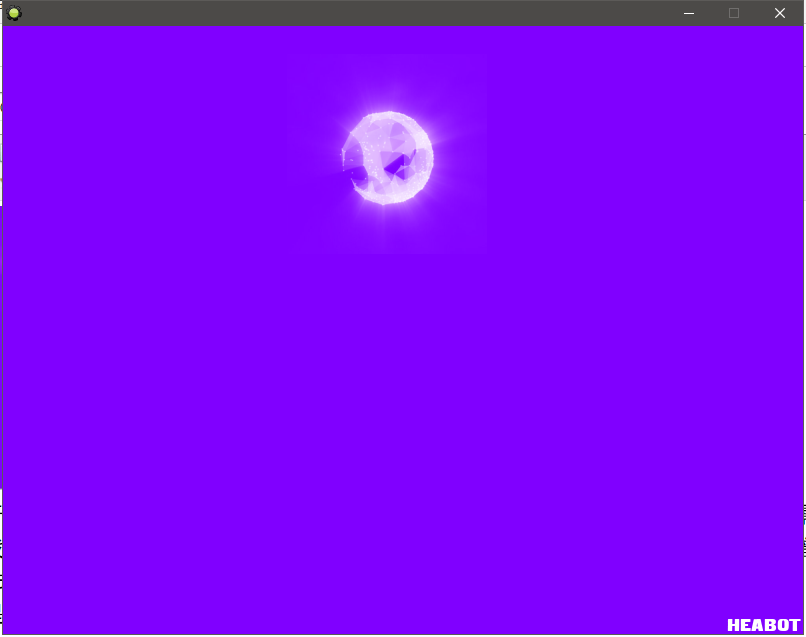### 区域反色效果

```1 2 3 4 5 draw_set_blend_mode_ext(bm_inv_dest_color, bm_zero); draw_set_color(c_white); draw_set_alpha(1); draw_rectangle(200, 200, 400, 400, false); // 这里可以改成绘制任何纯白色的图案或精灵 draw_set_blend_mode(bm_normal); ```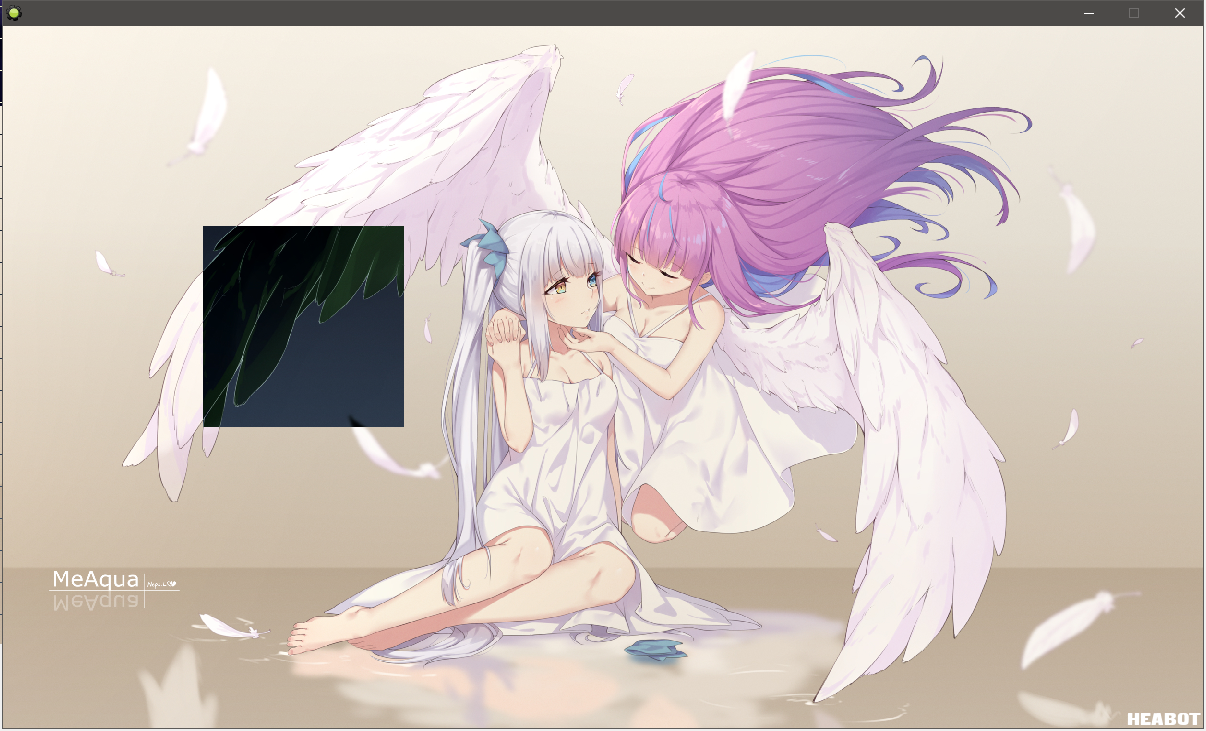```1 2 3 Ro = 1 - Rd Go = 1 - Gd Bo = 1 - Bd ```

Ro = 1 * (1 - Rd) + Rd * 0 = 1 - Rd

Go和Bo同理，不赘述。

## 绘制函数中的混色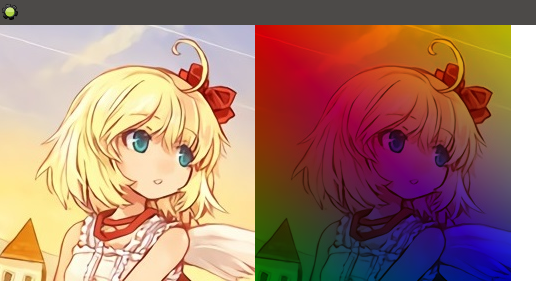```1 2 3 4 Ro = Rs * Rd Go = Gs * Gd Bo = Bs * Bd Ao = As * Ad // 实际上强制设置为1 ```

```1 2 3 4 5 6 7 8 9 10 11 12 13 14 // 左上角 draw_sprite(sprite_index, 0, 0, 0); // 右上角 draw_sprite_general(sprite_index, 0, 0, 0, sprite_width, sprite_height, sprite_width, 0, 1, 1, 0, c_red, c_yellow, c_blue, c_green, 1); // 左下角 draw_sprite(sprite_index, 0, 0, sprite_height); draw_set_blend_mode_ext(bm_dest_color, bm_zero); draw_rectangle_color(0, sprite_height, sprite_width, sprite_height * 2, c_red, c_yellow, c_blue, c_green, 0); draw_set_blend_mode(bm_normal); // 右下角 draw_sprite(sprite_index, 0, sprite_width, sprite_height); draw_set_blend_mode_ext(bm_zero, bm_src_color); draw_rectangle_color(sprite_width, sprite_height, sprite_width * 2, sprite_height * 2, c_red, c_yellow, c_blue, c_green, 0); draw_set_blend_mode(bm_normal); ```